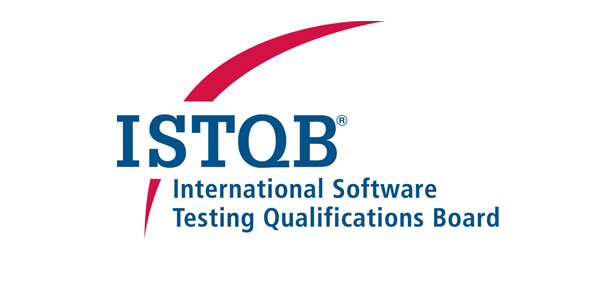# ISTQB Software Testing Quiz 5

6 Questions | Attempts: 3727
ShareSettingsISTQB software testing questions.

Take this ISTQB Software Testing Quiz 5 or create online test.

• 1.
Which of the following is not a quality characteristic listed in the ISO 9126 Standard?
• A.

Functionality

• B.

Usability

• C.

Supportability

• D.

Maintainability

• 2.
Boundary value testing...
• A.

... is the same as equivalence partition testing.

• B.

... tests values at the smallest incremental distance on either side of an equivalence partition boundary.

• C.

... tests combinations of input circumstances.

• D.

... is used in white box testing strategies.

• 3.
How many test cases are necessary to cover all the possible sequences of statements (paths) for the following program fragment?  Assume that the two conditions are independent of each other:IF (Condition 1)    THEN        Statement 1    ELSE        Statement 2ENDIF IF (Condition 2)THEN    Statement 3ENDIF
• A.

2 test cases

• B.

3 test cases

• C.

4 test cases

• D.

Not achievable

• 4.
In which order should tests be run?
• A.

The most important tests first.

• B.

The most difficult tests first (to get the hardest work finished early).

• C.

The easiest tests first (to give initial confidence).

• D.

The order they are thought of.

• 5.
Which of the following is the odd one out?
• A.

White box

• B.

Glass box

• C.

Structural

• D.

Functional

• 6.
A program validates a numeric field as follows: - Values less than 10 are rejected- Values between 10 and 21 are accepted- Values greater than or equal to 22 are rejected Which of the following input value sets covers all of the equivalence partitions?
• A.

10, 11, 21

• B.

3, 20, 21

• C.

3, 10, 22

• D.

10, 21, 22

## Related TopicsBack to top
×

Wait!
Here's an interesting quiz for you.